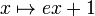Semidirect product need not preserve powering

Statement

It is possible to have a group$G$, a complemented normal subgroup$H$ (i.e.,$G$ is an internal semidirect product involving$H$), and a prime number$p$ such that the following hold:

•$H$ is powered over$p$.
• The quotient group$G/H$ (which is also isomorphic to any permutable complement to$H$ in$G$) is also powered over$p$.
•$G$ is not powered over$p$.

Note that it is not possible to construct finite examples, because in the finite case, being powered over a prime$p$ is equivalent to$p$ not dividing the order (see kth power map is bijective iff k is relatively prime to the order).

Proof

Below is an example where both$H$ and$G/H$ are rationally powered (i.e., powered over all primes), but$G$ is not powered over any prime. There may be simpler examples.

Let$W$ be the subgroup$\exp(\mathbb{Q})$ inside$(\R^*)^+$. Recall that the group GAPLus(1,R) is the group of affine maps from$\R$ to$\R$ where the multiplication is positive, i.e.:$GA^+(1,\R) = \R \rtimes (\R^*)^+$

Let$U$ be the vector space over$\mathbb{Q}$ generated by$W$ as a subset of$\R$. Note that$U$ is a subring of$\R$, because its generating set is a multiplicative monoid:$G = U \rtimes W$

Explicitly, it is the set of maps:$\{ x \mapsto ax + b \mid a \in W, b \in U \}$

Let$H$ be the base of the semidirect product here, so it is isomorphic to$U$.

•$H$ is powered over all primes: That's because it is isomorphic to$U$, a vector space over$\mathbb{Q}$.
•$G/H$ is powered over all primes: That is because it is isomorphic to$W = \exp(\mathbb{Q})$, which is isomorphic to$\mathbb{Q}$.
•$G$ is not powered over any prime. Consider the element of$G$ of the form$x \mapsto ex + 1$ (here,$e = \exp(1)$ is transcendental). For a prime$p$, the unique$p^{th}$ root of this in$GA^+(1,\R)$ is:$x \mapsto e^{1/p}x + \frac{1}{1 + e^{1/p} + e^{2/p} + \dots + e^{(p-1)/p}}$

We would like to claim that the number$\frac{1}{1 + e^{1/p} + e^{2/p} + \dots + e^{(p-1)/p}}$ is not an element of$U$, so that this$p^{th}$ root is not in$G$. This can be deduced from the fact that$e$ is trancendental.